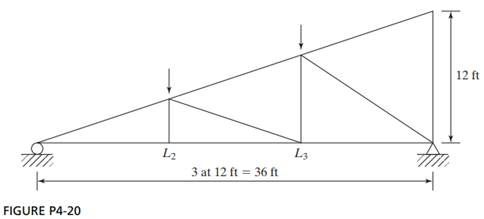Chat Now

# Design member L2L3 of the truss shown in Fig P420 It is to consist of a pair of angles with a in gusset plate between the angles at each joint Use A36 steel and assume two lines of three in

### Design member L2L3 of the truss shown in Fig P420 It is to consist of a pair of angles with a in gusset plate between the angles at each joint Use A36 steel and assume two lines of three in

Design member L2L3 of the truss shown in Fig. P4-20. It is to consist of a pair of angles with a -in gusset plate between the angles at each joint. Use A36 steel and assume two lines of three -in Ø bolts in each vertical angle leg, 4 in on center. Consider only the angles shown in the double-angle tables of the AISC Manual. For each load, PD = 60 k and PL = 48 k. Do not consider block shear.rahul 07-Jul-2020Get solution